Courses

# Potential Energy Mechanical Engineering Notes | EduRev

## Mechanical Engineering : Potential Energy Mechanical Engineering Notes | EduRev

The document Potential Energy Mechanical Engineering Notes | EduRev is a part of the Mechanical Engineering Course Engineering Mechanics - Notes, Videos, MCQs & PPTs.
All you need of Mechanical Engineering at this link: Mechanical Engineering

Potential Energy and Conservative Forces

Work is done by a force, and some forces, such as weight, have special characteristics. A conservative force is one, like the gravitational force, for which work done by or against it depends only on the starting and ending points of a motion and not on the path taken. We can define a potential energy (PE) for any conservative force, just as we did for the gravitational force. For example, when you wind up a toy, an egg timer, or an old-fashioned watch, you do work against its spring and store energy in it. (We treat these springs as ideal, in that we assume there is no friction and no production of thermal energy.) This stored energy is recoverable as work, and it is useful to think of it as potential energy contained in the spring. Indeed, the reason that the spring has this characteristic is that its force is conservative. That is, a conservative force results in stored or potential energy. Gravitational potential energy is one example, as is the energy stored in a spring. We will also see how conservative forces are related to the conservation of energy.

Potential Energy And Conservative Forces

Potential energy is the energy a system has due to position, shape, or configuration. It is stored energy that is completely recoverable.

A conservative force is one for which work done by or against it depends only on the starting and ending points of a motion and not on the path taken.

We can define a potential energy (PE) for any conservative force. The work done against a conservative force to reach a final configuration depends on the configuration, not the path followed, and is the potential energy added.

Potential Energy of a Spring

First, let us obtain an expression for the potential energy stored in a spring (PEs). We calculate the work done to stretch or compress a spring that obeys Hooke’s law. (Hooke’s law was examined in Elasticity: Stress and Strain, and states that the magnitude of force F on the spring and the resulting deformation ΔL are proportional, kΔL.) (See Figure 1.) For our spring, we will replace ΔL (the amount of deformation produced by a force F) by the distance x that the spring is stretched or compressed along its length. So the force needed to stretch the spring has magnitude Fkx, where k is the spring’s force constant. The force increases linearly from 0 at the start to kx in the fully stretched position. The average force is kx/2

Thus the work done in stretching or compressing the spring is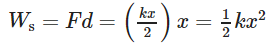Alternatively, we noted in Kinetic Energy and the Work-Energy Theorem that the area under a graph of F vs. x is the work done by the force. In Figure 1c we see that this area is also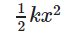We therefore define the potential energy of a spring, PEs, to be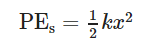where k is the spring’s force constant and x is the displacement from its undeformed position. The potential energy represents the work done on the spring and the energy stored in it as a result of stretching or compressing it a distance x. The potential energy of the spring PEs does not depend on the path taken; it depends only on the stretch or squeeze x in the final configuration.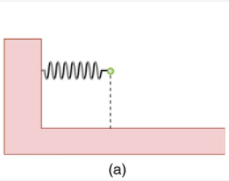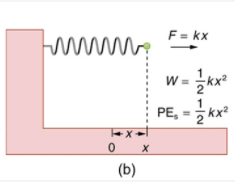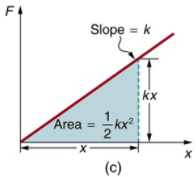Figure 1. (a) An undeformed spring has no PEs stored in it. (b) The force needed to stretch (or compress) the spring a distance x has a magnitude F = kx, and the work done to stretch (or compress) it isBecause the force is conservative, this work is stored as potential energy (PEs) in the spring, and it can be fully recovered. (c) A graph of F vs. x has a slope of k, and the area under the graph isThus the work done or potential energy stored isThe equation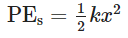has general validity beyond the special case for which it was derived. Potential energy can be stored in any elastic medium by deforming it. Indeed, the general definition of potential energy is energy due to position, shape, or configuration. For shape or position deformations, stored energy is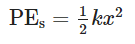where k is the force constant of the particular system and x is its deformation. Another example is seen in Figure 2 for a guitar string.

Conservation of Mechanical Energy

Let us now consider what form the work-energy theorem takes when only conservative forces are involved. This will lead us to the conservation of energy principle. The work-energy theorem states that the net work done by all forces acting on a system equals its change in kinetic energy. In equation form, this is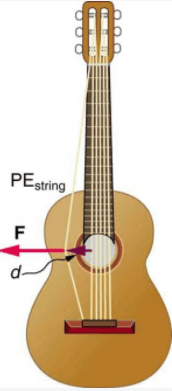Figure 2. Work is done to deform the guitar string, giving it potential energy. When released, the potential energy is converted to kinetic energy and back to potential as the string oscillates back and forth. A very small fraction is dissipated as sound energy, slowly removing energy from the string.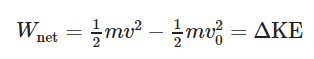If only conservative forces act, then Wnet = Wc, where Wc is the total work done by all conservative forces. Thus, Wc = ΔKE.

Now, if the conservative force, such as the gravitational force or a spring force, does work, the system loses potential energy. That is, Wc=−ΔPE. Therefore, −ΔPE = ΔKE or ΔKE + ΔPE = 0.

This equation means that the total kinetic and potential energy is constant for any process involving only conservative forces. That is,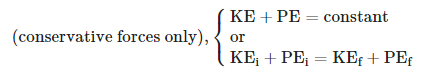where i and f denote initial and final values. This equation is a form of the work-energy theorem for conservative forces; it is known as the conservation of mechanical energy principle. Remember that this applies to the extent that all the forces are conservative, so that friction is negligible. The total kinetic plus potential energy of a system is defined to be its mechanical energy, (KE+PE). In a system that experiences only conservative forces, there is a potential energy associated with each force, and the energy only changes form between KE and the various types of PE, with the total energy remaining constant.

Example 1. Using Conservation of Mechanical Energy to Calculate the Speed of a Toy Car

A 0.100-kg toy car is propelled by a compressed spring, as shown in Figure 3. The car follows a track that rises 0.180 m above the starting point. The spring is compressed 4.00 cm and has a force constant of 250.0 N/m. Assuming work done by friction to be negligible, find the following:

1. How fast is the car going before it starts up the slope?
2. How fast is it going at the top of the slope?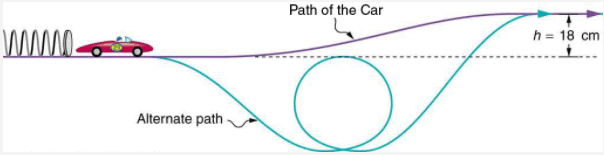Figure 3. A toy car is pushed by a compressed spring and coasts up a slope. Assuming negligible friction, the potential energy in the spring is first completely converted to kinetic energy, and then to a combination of kinetic and gravitational potential energy as the car rises. The details of the path are unimportant because all forces are conservative—the car would have the same final speed if it took the alternate path shown.

#### Strategy

The spring force and the gravitational force are conservative forces, so conservation of mechanical energy can be used. Thus,

KE+ PEi = KEf + PEf

or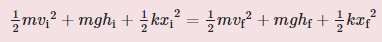where h is the height (vertical position) and x is the compression of the spring. This general statement looks complex but becomes much simpler when we start considering specific situations. First, we must identify the initial and final conditions in a problem; then, we enter them into the last equation to solve for an unknown.

#### Solution for Part 1

This part of the problem is limited to conditions just before the car is released and just after it leaves the spring. Take the initial height to be zero, so that both hi and hf are zero. Furthermore, the initial speed vi is zero and the final compression of the spring xf is zero, and so several terms in the conservation of mechanical energy equation are zero and it simplifies to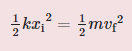In other words, the initial potential energy in the spring is converted completely to kinetic energy in the absence of friction. Solving for the final speed and entering known values yields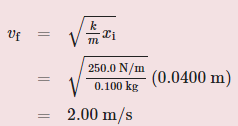#### Solution for Part 2

One method of finding the speed at the top of the slope is to consider conditions just before the car is released and just after it reaches the top of the slope, completely ignoring everything in between. Doing the same type of analysis to find which terms are zero, the conservation of mechanical energy becomes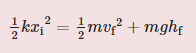This form of the equation means that the spring’s initial potential energy is converted partly to gravitational potential energy and partly to kinetic energy. The final speed at the top of the slope will be less than at the bottom. Solving for vf and substituting known values gives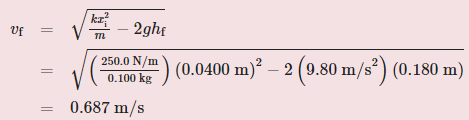Note that, for conservative forces, we do not directly calculate the work they do; rather, we consider their effects through their corresponding potential energies, just as we did in Example 1. Note also that we do not consider details of the path taken—only the starting and ending points are important (as long as the path is not impossible). This assumption is usually a tremendous simplification, because the path may be complicated and forces may vary along the way.

Offer running on EduRev: Apply code STAYHOME200 to get INR 200 off on our premium plan EduRev Infinity!

,

,

,

,

,

,

,

,

,

,

,

,

,

,

,

,

,

,

,

,

,

;